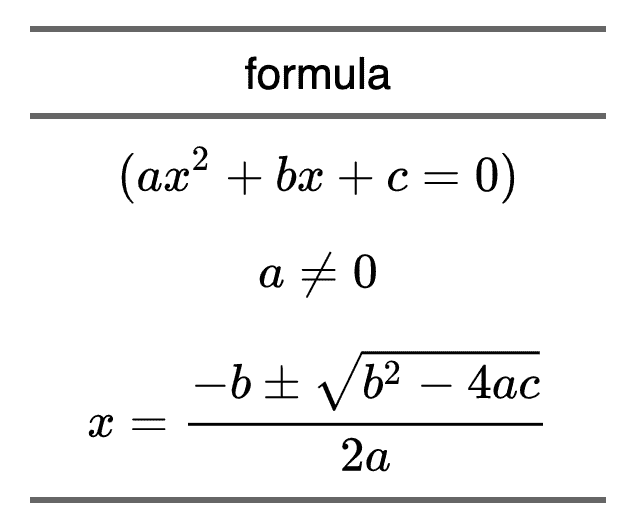# Insert equations

## Packages utilisés

We use the ‘equatiomatic’ package which will allow us to get the equation of the model in ‘latex’ format.

library(flextable)
library(equatiomatic)

## Le flextable

We will generate a flextable from a linear model using the function as_flextable().

mod <- lm(mpg ~ cyl + disp, mtcars)
ft <- as_flextable(mod)
ft
 Estimate Standard Error t value Pr(>|t|) (Intercept) 34.661 2.547 13.609 0.0000 *** cyl -1.587 0.712 -2.230 0.0337 * disp -0.021 0.010 -2.007 0.0542 . Signif. codes: 0 <= '***' < 0.001 < '**' < 0.01 < '*' < 0.05 Residual standard error: 3.055 on 29 degrees of freedom Multiple R-squared: 0.7596, Adjusted R-squared: 0.743 F-statistic: 45.81 on 29 and 2 DF, p-value: 0.0000

## Ajouter l’équation dans le tableau

The function equatiomatic::extract_eq() will be used to get the equation in latex format:

eq <- extract_eq(mod)
print(eq)
## $$## \operatorname{mpg} = \alpha + \beta_{1}(\operatorname{cyl}) + \beta_{2}(\operatorname{disp}) + \epsilon ##$$

It remains finally to add it in the table with the function flextable::compose(). You have to indicate that you want to treat the value as a ‘latex’ equation by using the function as_equation().

ft <- add_header_lines(ft, "", top = TRUE)

ft <- compose(
x = ft, j = 1, i = 1, part = "header",
value = as_paragraph(
as_equation(eq,
width = 2, height = .5)
)
)

ft <- align(ft, i = 1, part = "header", align = "center")
ft
 $\operatorname{mpg} = \alpha + \beta_{1}(\operatorname{cyl}) + \beta_{2}(\operatorname{disp}) + \epsilon$ Estimate Standard Error t value Pr(>|t|) (Intercept) 34.661 2.547 13.609 0.0000 *** cyl -1.587 0.712 -2.230 0.0337 * disp -0.021 0.010 -2.007 0.0542 . Signif. codes: 0 <= '***' < 0.001 < '**' < 0.01 < '*' < 0.05 Residual standard error: 3.055 on 29 degrees of freedom Multiple R-squared: 0.7596, Adjusted R-squared: 0.743 F-statistic: 45.81 on 29 and 2 DF, p-value: 0.0000

## Another example

eqs <- c(
"(ax^2 + bx + c = 0)",
"a \\ne 0",
"x = {-b \\pm \\sqrt{b^2-4ac} \\over 2a}")
df <- data.frame(formula = eqs)
df
##                                   formula
## 1                     (ax^2 + bx + c = 0)
## 2                                a \\ne 0
## 3 x = {-b \\pm \\sqrt{b^2-4ac} \\over 2a}

ft <- flextable(df)
ft <- compose(
x = ft, j = "formula",
value = as_paragraph(as_equation(formula, width = 2, height = .5)))
ft <- align(ft, align = "center", part = "all")
ft <- width(ft, width = 2)
ft
 formula $(ax^2 + bx + c = 0)$ $a \ne 0$ $x = {-b \pm \sqrt{b^2-4ac} \over 2a}$